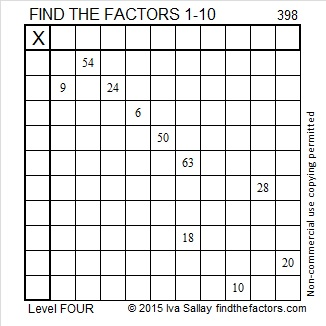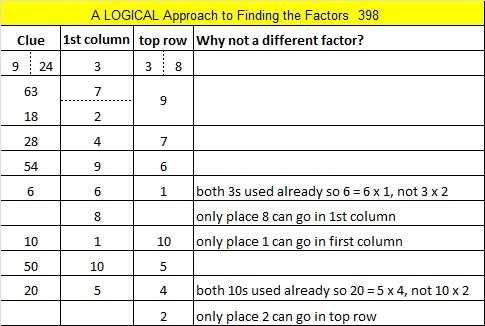# 398 and Level 4

Let’s apply a couple of divisibility rules to the number 398 to find some of its factors. 2 is a factor of 398 because 8 is even. 4 is NOT a factor of 398 because 4 is NOT a factor of 98. We know this because 8 is divisible by 4, and 9 is an odd number.Print the puzzles or type the factors on this excel file: 10 Factors 2015-02-16

• 398 is a composite number.
• Prime factorization: 398 = 2 x 199
• The exponents in the prime factorization are 1 and 1. Adding one to each and multiplying we get (1 + 1)(1 + 1) = 2 x 2 = 4. Therefore 398 has exactly 4 factors.
• Factors of 398: 1, 2, 199, 398
• Factor pairs: 398 = 1 x 398 or 2 x 199
• 398 has no square factors that allow its square root to be simplified. √398 ≈ 19.9499This site uses Akismet to reduce spam. Learn how your comment data is processed.